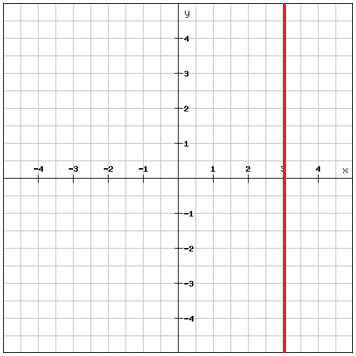# Writing linear equations using the point-slope form and the standard form

There are other ways to write the linear equation of a straight line than the slope-intersect form previously described

Example

We've got a line with the slope 2. One of the points that the line passes through has got the coordinates (3, 5). It's possible to write an equation relating x and y using the slope formula with

$\left (x _{1}\, ,y_{1} \right )=\left ( 3,5 \right )\: and\: \left ( x_{2},\, y_{2} \right )=\left ( x,y \right )$

$m=\frac{y_{2}-y_{1}}{x_{2}-x_{1}}$

$2=\frac{y-5}{x-3}$

$2\, {\color{green} {\cdot \, \left ( x-3 \right )}}=\frac{\left ( y-5 \right )\, {\color{green} {\cdot \, \left ( x-3 \right )}}}{x-3}$

$2\left ( x-3 \right )=y-5$

Since we used the coordinates of one known point and the slope to write this form of equation it is called the point-slop form

$y-y_{1}=m\left ( x-x_{1} \right )$

Another way of writing linear equations is to use the standard form

$Ax+By=C$

Where A, B and C are real numbers and where A and B are not both zero.

Since the slope of a vertical line is undefined you can't write the equation of a vertical line using neither the slope-intersect form or the point-slope form. But you can express it using the standard form.

Example

Write the equation of the lineFor any given point of the line the x-coordinate is 3. This means that the equation of the line is

$x=3$

## Video lesson

Write the linear equation in the point-slope form for the line that passes through (-1, 4) and has a slope of -1